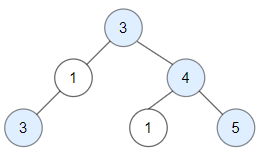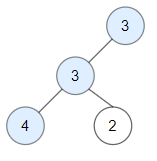1448. Count Good Nodes in Binary Tree
Medium
4.9K
128

Given a binary tree `root`, a node X in the tree is named good if in the path from root to X there are no nodes with a value greater than X.

Return the number of good nodes in the binary tree.

Example 1:```Input: root = [3,1,4,3,null,1,5]
Output: 4
Explanation: Nodes in blue are good.
Root Node (3) is always a good node.
Node 4 -> (3,4) is the maximum value in the path starting from the root.
Node 5 -> (3,4,5) is the maximum value in the path
Node 3 -> (3,1,3) is the maximum value in the path.```

Example 2:```Input: root = [3,3,null,4,2]
Output: 3
Explanation: Node 2 -> (3, 3, 2) is not good, because "3" is higher than it.```

Example 3:

```Input: root = 
Output: 1
Explanation: Root is considered as good.```

Constraints:

• The number of nodes in the binary tree is in the range `[1, 10^5]`.
• Each node's value is between `[-10^4, 10^4]`.
Accepted
325.8K
Submissions
439.4K
Acceptance Rate
74.1%

Seen this question in a real interview before?
1/4
Yes
No

Discussion (0)

Related Topics